# Ppt on triangles class x made my jatin jangid

12. Sep 2021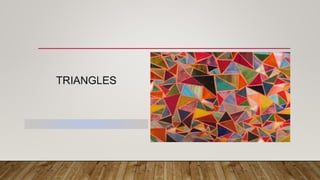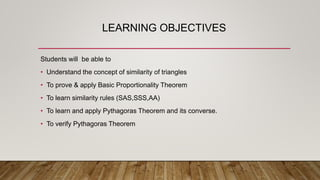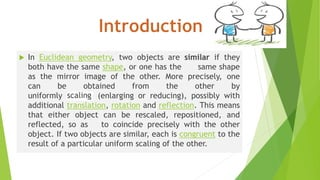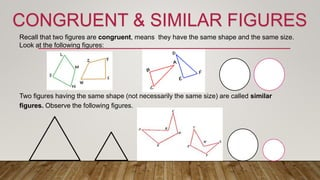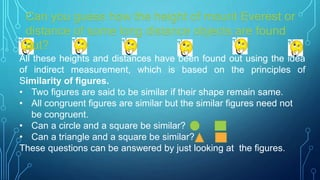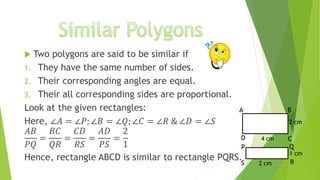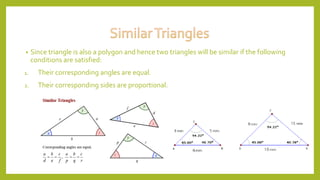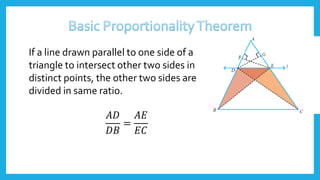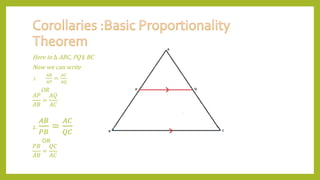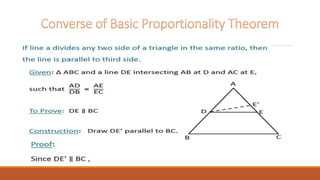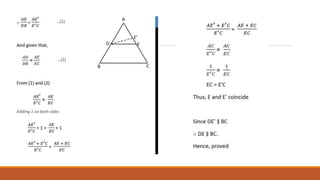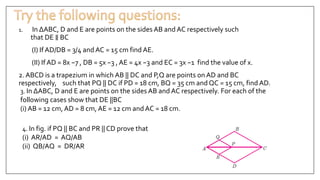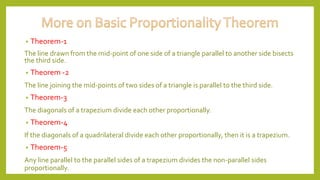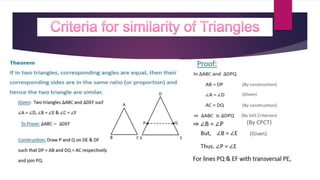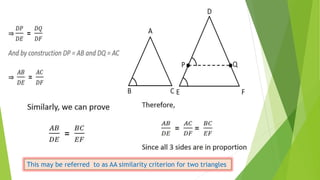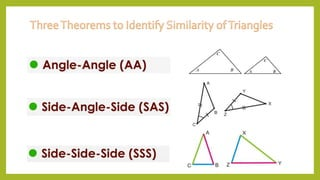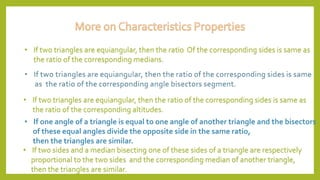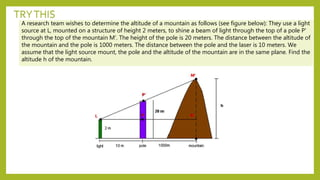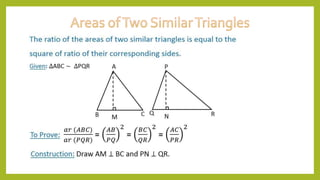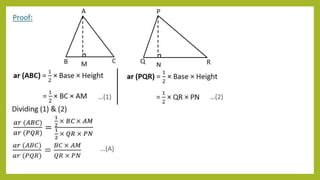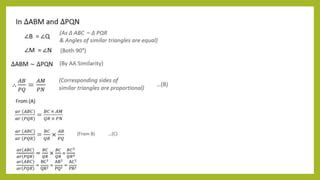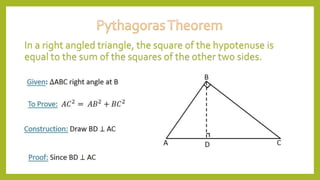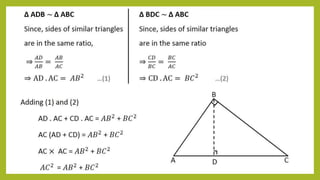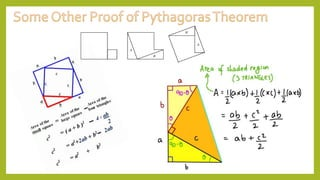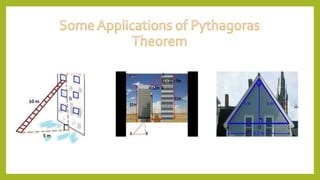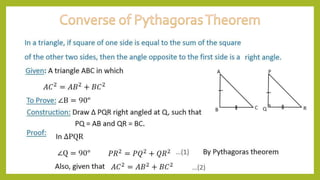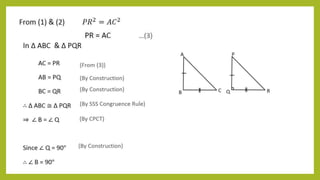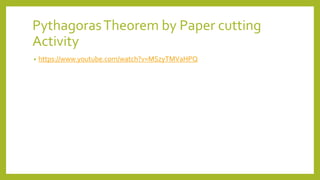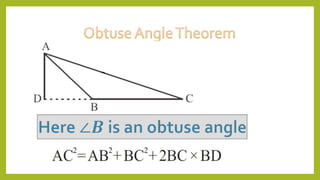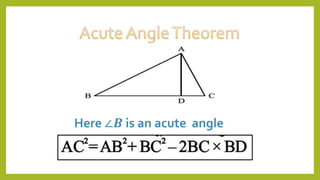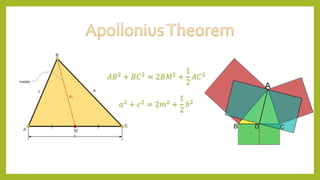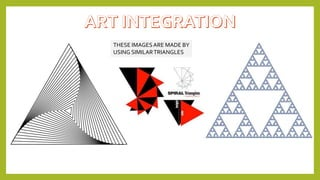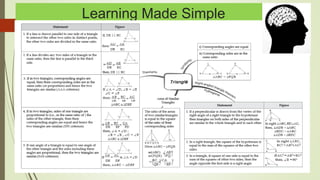1 von 34

### Ppt on triangles class x made my jatin jangid

• 2. LEARNING OBJECTIVES Students will be able to • Understand the concept of similarity of triangles • To prove & apply Basic Proportionality Theorem • To learn similarity rules (SAS,SSS,AA) • To learn and apply Pythagoras Theorem and its converse. • To verify Pythagoras Theorem
• 3.  In Euclidean geometry, two objects are similar if they both have the same shape, or one has the same shape as the mirror image of the other. More precisely, one can be obtained from the other by uniformly (enlarging or reducing), possibly with additional translation, rotation and reflection. This means that either object can be rescaled, repositioned, and reflected, so as to coincide precisely with the other object. If two objects are similar, each is congruent to the result of a particular uniform scaling of the other.
• 4. Recall that two figures are congruent, means they have the same shape and the same size. Look at the following figures: Two figures having the same shape (not necessarily the same size) are called similar figures. Observe the following figures. CONGRUENT & SIMILAR FIGURES
• 5. All these heights and distances have been found out using the idea of indirect measurement, which is based on the principles of Similarity of figures. • Two figures are said to be similar if their shape remain same. • All congruent figures are similar but the similar figures need not be congruent. • Can a circle and a square be similar? • Can a triangle and a square be similar? These questions can be answered by just looking at the figures. Can you guess how the height of mount Everest or distance of some long distance objects are found out?
• 6.  Two polygons are said to be similar if 1. They have the same number of sides. 2. Their corresponding angles are equal. 3. Their all corresponding sides are proportional. Look at the given rectangles: Here, ∠𝐴 = ∠𝑃; ∠𝐵 = ∠𝑄; ∠𝐶 = ∠𝑅 & ∠𝐷 = ∠𝑆 𝐴𝐵 𝑃𝑄 = 𝐵𝐶 𝑄𝑅 = 𝐶𝐷 𝑅𝑆 = 𝐴𝐷 𝑃𝑆 = 2 1 Hence, rectangle ABCD is similar to rectangle PQRS. A B C D S R P Q 4 cm 2 cm 1 cm 2 cm
• 7. • Since triangle is also a polygon and hence two triangles will be similar if the following conditions are satisfied: 1. Their corresponding angles are equal. 2. Their corresponding sides are proportional.
• 8. If a line drawn parallel to one side of a triangle to intersect other two sides in distinct points, the other two sides are divided in same ratio. 𝐴𝐷 𝐷𝐵 = 𝐴𝐸 𝐸𝐶
• 9. Here in ∆ ABC, PQ ∥ BC Now we can write 1. 𝐴𝐵 𝐴𝑃 = 𝐴𝐶 𝐴𝑄 OR 𝐴𝑃 𝐴𝐵 = 𝐴𝑄 𝐴𝐶 2. 𝐴𝐵 𝑃𝐵 = 𝐴𝐶 𝑄𝐶 OR 𝑃𝐵 𝐴𝐵 = 𝑄𝐶 𝐴𝐶
• 12. 1. In ΔABC, D and E are points on the sides AB and AC respectively such that DE ∥ BC (I) If AD/DB = 3/4 and AC = 15 cm find AE. (II) If AD = 8x −7 , DB = 5x −3 , AE = 4x −3 and EC = 3x −1 find the value of x. 2. ABCD is a trapezium in which AB || DC and P,Q are points on AD and BC respectively, such that PQ || DC if PD = 18 cm, BQ = 35 cm and QC = 15 cm, find AD. 3. In ΔABC, D and E are points on the sides AB and AC respectively. For each of the following cases show that DE ||BC (i) AB = 12 cm, AD = 8 cm, AE = 12 cm and AC = 18 cm. 4. In fig. if PQ || BC and PR || CD prove that (i) AR/AD = AQ/AB (ii) QB/AQ = DR/AR
• 13. • Theorem-1 The line drawn from the mid-point of one side of a triangle parallel to another side bisects the third side. • Theorem -2 The line joining the mid-points of two sides of a triangle is parallel to the third side. • Theorem-3 The diagonals of a trapezium divide each other proportionally. • Theorem-4 If the diagonals of a quadrilateral divide each other proportionally, then it is a trapezium. • Theorem-5 Any line parallel to the parallel sides of a trapezium divides the non-parallel sides proportionally.
• 15. This may be referred to as AA similarity criterion for two triangles
• 17. • If two triangles are equiangular, then the ratio Of the corresponding sides is same as the ratio of the corresponding medians. • If two triangles are equiangular, then the ratio of the corresponding sides is same as the ratio of the corresponding altitudes. • If one angle of a triangle is equal to one angle of another triangle and the bisectors of these equal angles divide the opposite side in the same ratio, then the triangles are similar. • If two sides and a median bisecting one of these sides of a triangle are respectively proportional to the two sides and the corresponding median of another triangle, then the triangles are similar.
• 18. A research team wishes to determine the altitude of a mountain as follows (see figure below): They use a light source at L, mounted on a structure of height 2 meters, to shine a beam of light through the top of a pole P' through the top of the mountain M'. The height of the pole is 20 meters. The distance between the altitude of the mountain and the pole is 1000 meters. The distance between the pole and the laser is 10 meters. We assume that the light source mount, the pole and the altitude of the mountain are in the same plane. Find the altitude h of the mountain. TRYTHIS
• 21. 𝑎𝑟 𝐴𝐵𝐶 𝑎𝑟 𝑃𝑄𝑅 = 𝐵𝐶 𝑄𝑅 × 𝐵𝐶 𝑄𝑅 = 𝐵𝐶2 𝑄𝑅2 𝑎𝑟 𝐴𝐵𝐶 𝑎𝑟 𝑃𝑄𝑅 = BC2 QR2 = AB2 PQ2 = AC2 PR2
• 22. In a right angled triangle, the square of the hypotenuse is equal to the sum of the squares of the other two sides.
• 28. PythagorasTheorem by Paper cutting Activity • https://www.youtube.com/watch?v=MSzyTMVaHPQ
• 30. Here ∠𝑩 is an acute angle
• 31. 𝐴𝐵2 + 𝐵𝐶2 = 2𝐵𝑀2 + 1 2 𝐴𝐶2 𝑎2 + 𝑐2 = 2𝑚2 + 1 2 𝑏2
• 32. THESE IMAGESARE MADE BY USING SIMILARTRIANGLES
• 33. Learning Made Simple e